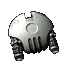## subset example, removing parentheses from type names

This is a moderated forum that collects tutorials, guides, and references for creating Transcendence extensions and scripts.
relanat
Militia CaptainPosts: 703
Joined: Tue Nov 05, 2013 9:56 am
The function help for 'subset' is this:

Code: Select all

``````(subset list pos [count]) -> list
``````
The function works for strings as well.

Code: Select all

``````(subset string pos [count]) -> string
``````
It is a very well named function because it does indeed take a subset.
The numbering system used is 0-based. This means that the function counts from 0, not 1, for the first character.
This can be a little confusing.

Some types have a name which is enclosed in brackets. These names weren't really meant for use in the game, but when has that ever stopped a modder!
An example. Enter the first line of code into the debug console and press 'Enter'. The name "(Commonwealth metropolis)" is printed to the console. 1056779 is the decimal UNID equivalent of &stCommonwealthStation; which is the Startons in SOTP (except Starton Eridani which is a separate 'StationType').

Code: Select all

``````(setq theTypeName (typGetProperty 1056779 'name))
(Commonwealth metropolis)
``````
We will use 'subset' to remove the brackets and leave the name as "Commonwealth metropolis".

Type the first line of this code into the console and press 'Enter'.

Code: Select all

``````(subset theTypeName 0 1)
(
``````
What have we done here? The code is taking a subset of 'theTypeName', EDIT: "(Commonwealth Station)""(Commonwealth metropolis)", which starts at 'pos'ition 0 and is 1 count long, so one character starting at the beginning of the name. This is '(' as shown in the console.
The 'pos' value is 0 which, because of the 0-based numbering system, is the beginning of the name.
The 'count' value, which is optional as indicated by the square brackets '[' and ']' in the function help, is 1 so the subset is only one character long.

We can check if the first character of 'theTypeName' is '(' using this code.

Code: Select all

``````(eq (subset theTypeName 0 1) "(")
True
``````
The first character is 'eq'ual to '(' so the debug console prints 'True'.

How do we check if the last character is ')'?
First we use 'count' to get the number of characters in 'theTypeName'.

Code: Select all

``````(setq typeNameCount (count theTypeName))
25
``````
This is how many letters are in the name as well as the space between the two words and the two brackets. Total: 25.
So getting a subset of 'theTypeName' at 'pos' 25 with a count of '1' would seem to be the required code. But it doesn't work.

Code: Select all

``````(subset theTypeName 25 1)
Nil
``````
'count' uses 1-based numbering the same as normal human-type counting. So it starts at number 1 for the first character, 2 for the second, 3 for the third, etc.Because 'subset' uses 0-based numbering, not starting at 1 as we humans normally do, we need to change the value to one less.
Because 0-based numbering sees the first character in the string as 'pos' 0, the second character as 'pos' 1, the third as 'pos' 2, etc, to get the twenty-fifth character, you guessed it, we use 'pos' 24.

Code: Select all

``````(subset theTypeName 24 1)
)
``````
To do this automatically for names of different lengths we can use this code:

Code: Select all

``````(subset theTypeName (subtract typeNameCount 1) 1)
)
``````
This takes a subset of 'theTypeName' at a 'pos' of one less than the count of the name which is what we need.

Okay. So now we know how to check if the first and last characters are brackets.
Since the brackets are there we now want to remove them.
This involves taking a subset of all the characters from pos 1, the second character in the name, to the second to last character.

Code: Select all

``````(setq noBracketsTypeName (subset theTypeName 1 (subtract typeNameCount 2)))
Commonwealth metropolis
``````
What this code does is take a subset from 'pos' 1 for a 'count' of 2 less than the length of the name.
In this example, 'pos' 1 is the capital C in "Commonwealth", the second character in the name, and the count is 23 which finishes at the 's' at the end of metropolis. So the brackets get removed.

All together we would use:

Code: Select all

``````(block Nil
(setq theTypeName (typGetProperty 1056779 'name))
(setq typeNameCount (count theTypeName))
(setq noBracketsTypeName
(if (and (eq (subset theTypeName 0 1) "(")
(eq (subset theTypeName (subtract typeNameCount 1) 1) ")")
)
(subset theTypeName 1 (subtract typeNameCount 2))
theTypeName
)
)
)
``````
This checks for brackets as the first and last characters in the name and if the brackets are there it removes them.
If the name doesn't have brackets it just shows the name as normal.
Other examples to try are decimal UNID 983045, &stWeaponsCache;, which has brackets in its name and 1056775 which is &stKorolovShipping; which doesn't. Even though names may or may not have brackets around their names and be of different lengths the code handles this automatically.